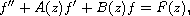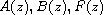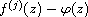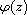Electron. J. Diff. Equ., Vol. 2015 (2015), No. 68, pp. 1-12.

### Oscillation of arbitrary-order derivatives of solutions to linear differential equations taking small functions in the unit disc Pan Gong, Li-Peng Xiao

Abstract:
In this article, we study the relationship between solutions and their derivatives of the differential equationwhereare meromorphic functions of finite iterated p-order in the unit disc. We obtain some oscillation theorems for, where f is a solution andis a small function.

Submitted January 6, 2015. Published March 20, 2015.
Math Subject Classifications: 34M10, 30D35.
Key Words: Unit disc; iterated order; growth; exponent of convergence.

Show me the PDF file (236 KB), TEX file, and other files for this article.Pan Gong Institute of Mathematics and Information Science Jiangxi Normal University Nanchang 330022, China email: gongpan12@163.com Li Peng Xiao Institute of Mathematics and Information Science Jiangxi Normal University Nanchang 330022, China email: 2992507211@qq.com